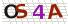2021-07-10 |

# Python 数字求和

```# 用户输入数字
num1 = 6
num2 = input('输入第一个数字：')

# 求和
sum =num1 + float(num2)

# 显示计算结果
print(
'数字 {0} 和 {1} 相加结果为： {2}'
.format(num1, num2, sum)
)
```

```输入第一个数字：8

```

```print(
'两数之和为 %.1f'
%(6+float(input('输入一个数字：'))))
```

### 发表评论

评价:
验证码:最新评论